# Maths - Euler Angles

Thanks very much to Andy for sending this to me, I will work though this to update the site. - MJB

Martin,

I've worked through the Quaternion and Euler angle sections of your rotation math pages in detail. I now have working C code written from scratch, so I'm pretty confident I understand what's going on. Your web pages have been hugely useful in helping me learn this stuff. However, in working through your material, I've found some errors and inconsistencies, particularly on the Euler Angles page. Feel free to publish this material however you see fit.My quaternion results match yours, so I'll start there. This picture is my interpretation of the NASA Standard Aeroplane coordinate system. I observe that this coordinate system is consistently left-handed. (I have trouble with the three finger rule, because I can never remember which way to point the fingers. For me the curled finger rule works well: when you point the thumb along the Z axis the fingers curl from X toward Y.) The rotations are also left-handed: point the left thumb along the rotation axis and the curled fingers point in the direction of the rotation: ψ= heading, + left θ= attitude, + up φ= bank, + left

From this picture I can develop the quaternions as a function of the Euler angles. You might consider adding this derivation to the Euler to Quaternion page https://www.euclideanspace.com/maths/geometry/rotations/conversions/eulerToQuaternion/index.htm .

From the basic definition of a quaternion, we can easily express the quaternion form for rotation along the X, Y, and Z axes, representing bank, attitude, and heading, respectively. Recall that the rotation quaternion (w, x, y, z) for the angle q is encoded as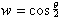and (x, y, z) represents a vector of lengthalong the axis of rotation. Note also that the handedness of the rotation must agree with the handedness of the coordinate system for the quaternion to be valid. Then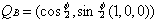To express the rotation of an arbitrary set of Euler angles ψ, θ, and φ we compose the rotations about the three axes by multiplying the quaternions. In the NASA standard aeroplane, the rotations are applied in the order heading, attitude, and bank. However, because each rotation is expressed in the frame of reference of the aircraft, from an outside frame of reference they must be composed in the reverse order. Remember that multiple quaternions are applied right to left:Substituting and multiplying out, we getwhich agrees with your result. I also come up with the same equivalence between quaternion and rotation matrix that you present on https://www.euclideanspace.com/maths/geometry/rotations/conversions/quaternionToMatrix/index.htm

I am also in agreement on the matrix notation and matrix/vector multiplications used on this page. I do note that in the second expansion of q * P1 * q' the signs of most of the terms in the first line are reversed. As written, the terms do not cancel to zero. This line should read

- qx*qw*x - qy*qw*y- qz*qw*z + qw*qx*x + qy*qx*z - qz*qx*y + qw*qy*y - qx*qy*z + qz*qy*x + qw*qz*z + qx*qz*y - qy*qz*x

Skipping ahead, my derivation of the conversion of quaternion to Euler angles also agrees with your result on https://www.euclideanspace.com/maths/geometry/rotations/conversions/quaternionToEuler/index.htm

All this is to set the stage for arguing that your presentation of Euler angles for the NASA standard aeroplane coordinate system on https://www.euclideanspace.com/maths/geometry/rotations/euler/index.htm

is incorrect or at least inconsistent with the above pages.

First, the basic rotation matrices are all transposed. Per the above quaternionToMatrix page, the matrix multiplication is done asorso thatThat means the basic rotation matrices should beSecond, your composition of the matrices is reversed. As I observed earlier, each rotation is expressed in the frame of reference of the aircraft, so that the rotations must be composed in the order. The matrices multiply out as follows: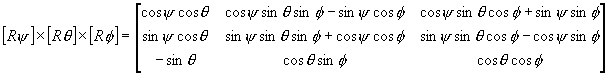The matrix given in Java code on

https://www.euclideanspace.com/maths/geometry/rotations/conversions/eulerToMatrix/index.htm

is also transposed, relative to the quaternionToMatrix page.

Comparing the matrix derived above to the matrix expressed in the quaternion component terms from the quaternionToMatrix page, we can derive the quaternion to Euler transformations on your quaternionToEuler page:so thatLikewise,soandsoThese transformations are fully self-consistent and can be verified by  substituting the Euler angle expressions for the quaternion components. For example, take the expression for q: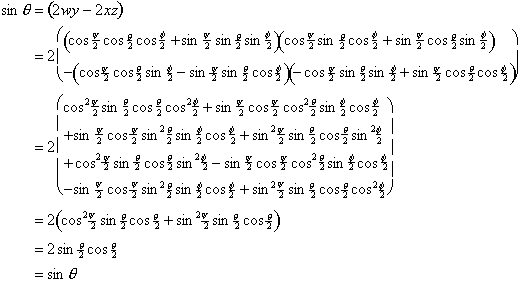Similar, but messier, verifications can be done for the other two angles: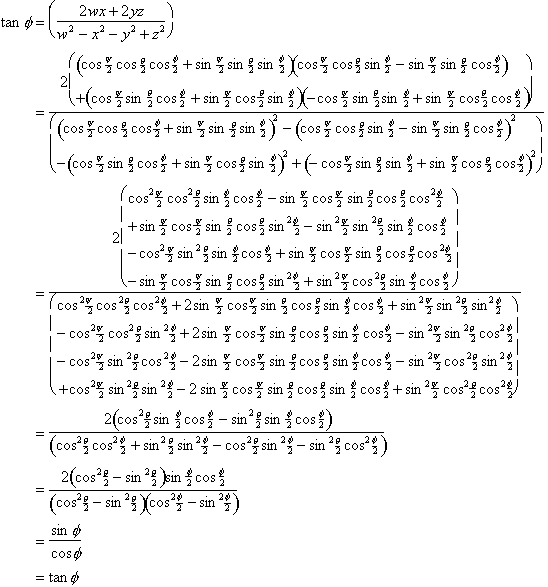metadata block see also: Correspondence about this page Book Shop - Further reading. Where I can, I have put links to Amazon for books that are relevant to the subject, click on the appropriate country flag to get more details of the book or to buy it from them.3D Math Primer - Aimed at complete beginners to vector and matrix algebra. Other Math Books

This site may have errors. Don't use for critical systems.